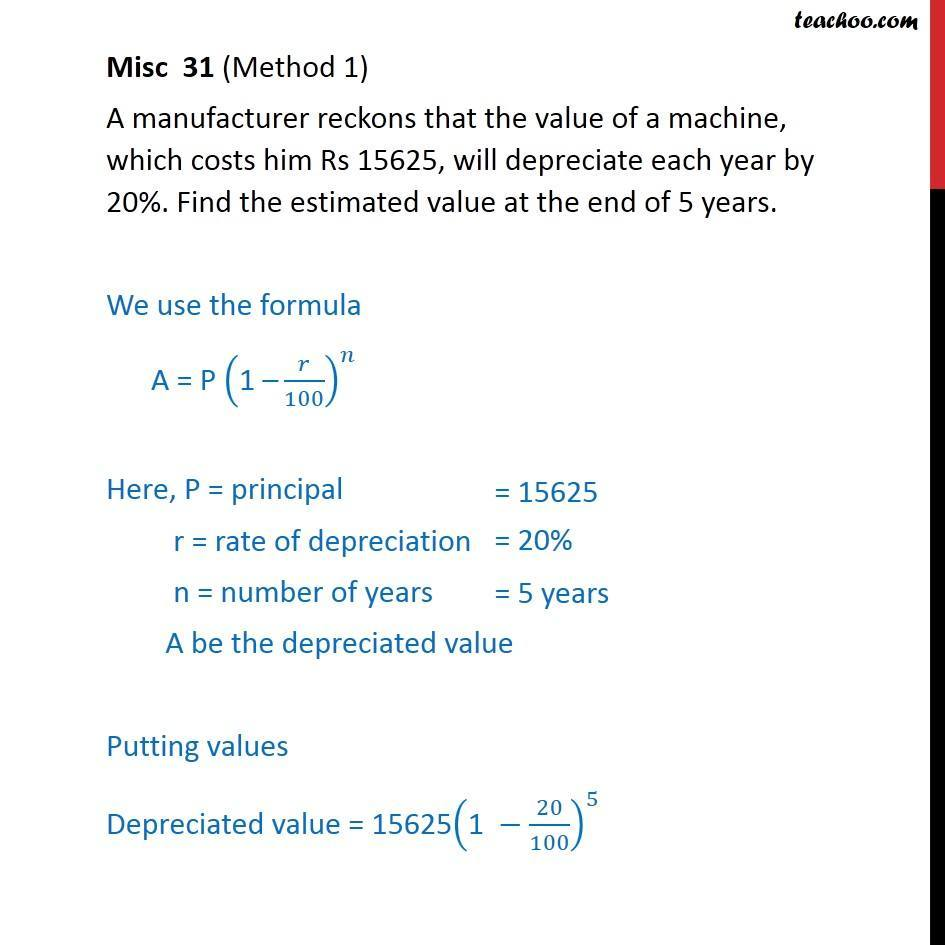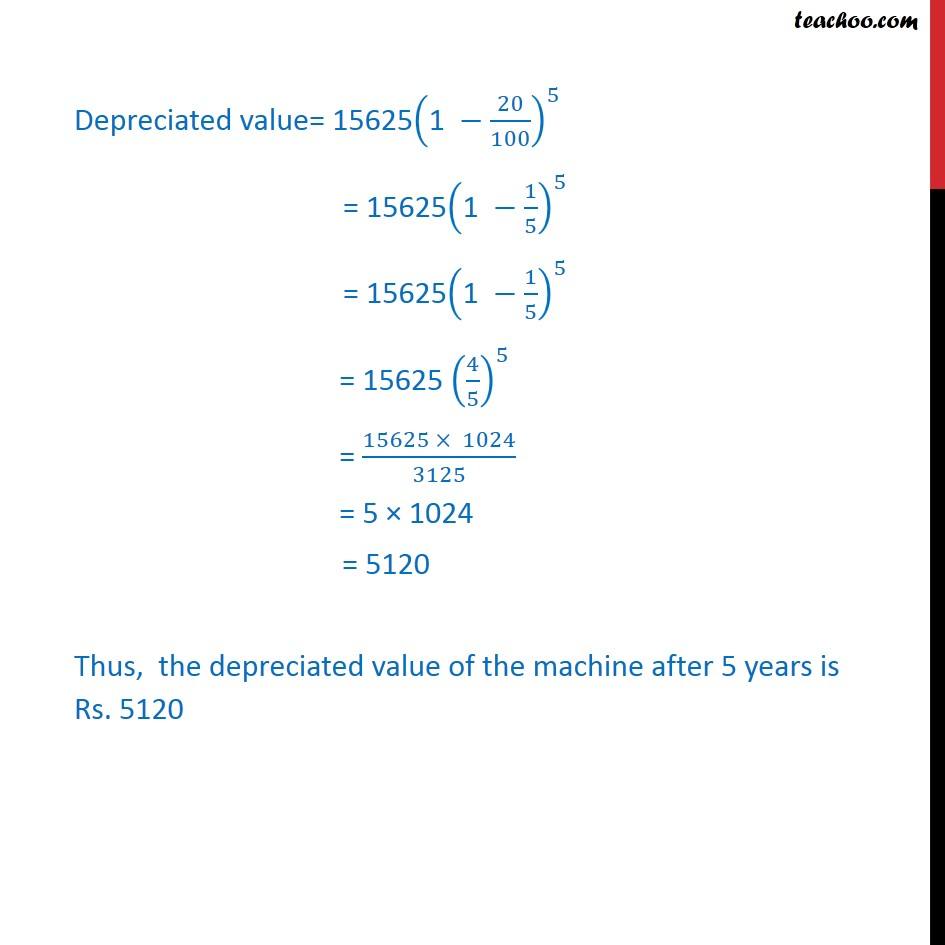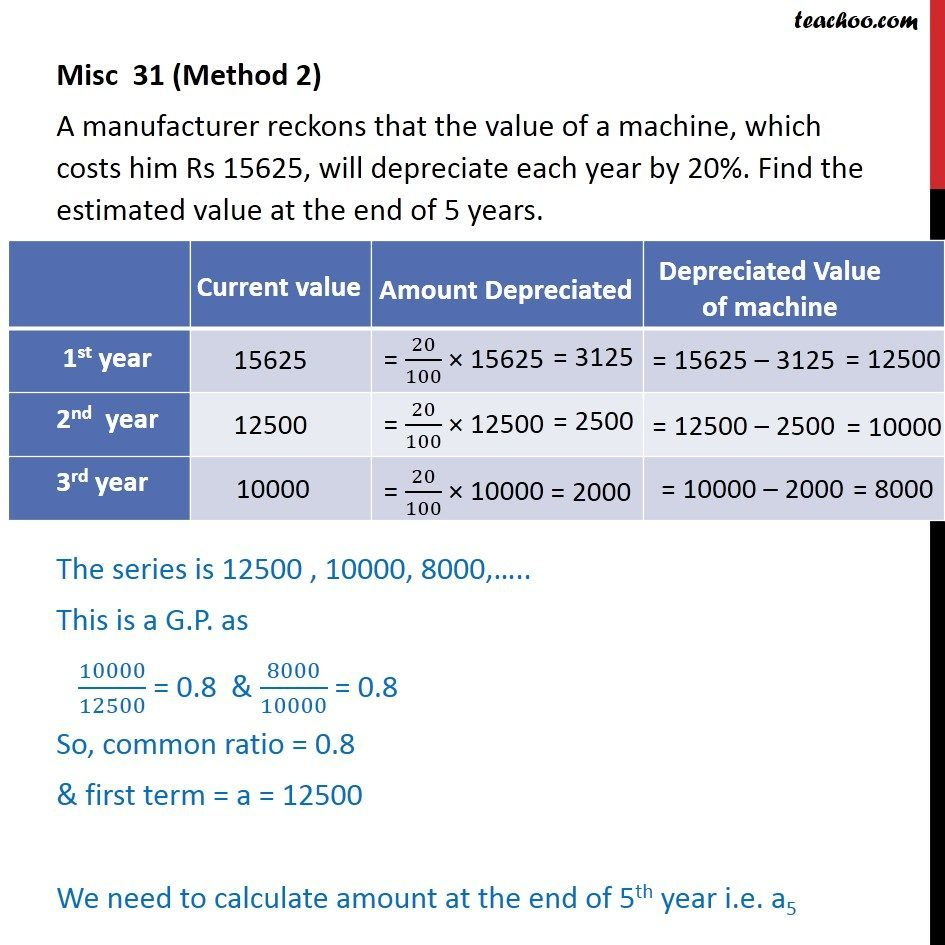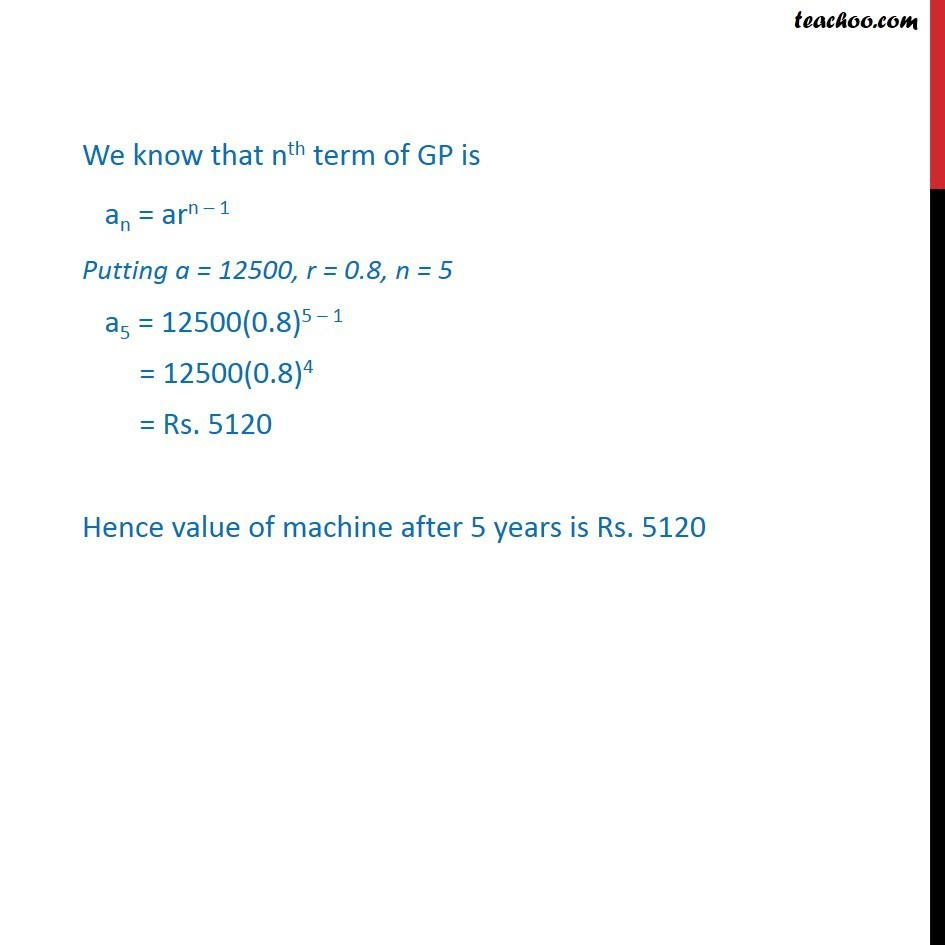Miscellaneous

Chapter 9 Class 11 Sequences and Series (Term 1)
Serial order wise641 students have Teachoo Black. What are you waiting for?

### Transcript

Misc 31 (Method 1) A manufacturer reckons that the value of a machine, which costs him Rs 15625, will depreciate each year by 20%. Find the estimated value at the end of 5 years. We use the formula A = P ("1 –" 𝑟/100)^𝑛 Here, P = principal r = rate of depreciation n = number of years A be the depreciated value Putting values Depreciated value = 15625("1 " −20/100)^5 = 15625("1 " −1/5)^5 = 15625("1 " −1/5)^5 = 15625 (4/5)^5 = (15625 × 1024)/3125 = 5 × 1025 = 5125 Thus, the depreciated value of the machine after 5 years is Rs. 5125 Misc 31 (Method 2) A manufacturer reckons that the value of a machine, which costs him Rs 15625, will depreciate each year by 20%. Find the estimated value at the end of 5 years. The series is 12500 , 10000, 8000,….. This is a G.P. as 10000/12500 = 0.8 & 8000/10000 = 0.8 So, common ratio = 0.8 & first term = a = 12500 We need to calculate amount at the end of 5th year i.e. a5 We know that nth term of GP is an = arn – 1 Putting a = 12500, r = 0.8, n = 5 a5 = 12500(0.8)5 – 1 = 12500(0.8)4 = Rs. 5120 Hence value of machine after 5 years is Rs. 5120